Courses

# Biasing FET Electrical Engineering (EE) Notes | EduRev

## Electronic Devices

Created by: Cstoppers Instructors

## Electrical Engineering (EE) : Biasing FET Electrical Engineering (EE) Notes | EduRev

The document Biasing FET Electrical Engineering (EE) Notes | EduRev is a part of the Electrical Engineering (EE) Course Electronic Devices.
All you need of Electrical Engineering (EE) at this link: Electrical Engineering (EE)

BIASING FET:-

For the proper functioning of a linear FET amplifier, it is necessary to maintain the operating point Q stable in the central portion of the pinch off region. The Q point should be independent of device parameter variations and ambient temperature variations.

This can be achieved by suitably selecting the gate to source voltage VGS and drain current ID which is referred to as biasing

JFET biasing circuits are very similar to BJT biasing circuits. The main difference between JFET circuits and BJT circuits is the operation of the active components themselves.

There are mainly two types of Biasing circuits

1. Self bias
2. Voltage divider bias.

7.3.1. SELF BIAS:-

Self bias is a JFET biasing circuit that uses a source resistor to help reverse bias the JFET gate.

A  self bias circuit is shown in the fig 7.3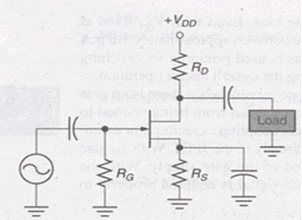Self bias is the most common type of JFET bias. This JFET must be operated such that gate source junction is always reverse biased. This condition requires a negative VGS for an N-channel JFET and a positive VGS for P-channel JFET. This can be achieved using the self bias arrangement as shown in Fig 7.3. The gate resistor RG doesn’t affect the bias because it has essentially no voltage drop across it, and the gate remains at 0V. RG is necessary only to isolate an ac signal from ground in amplifier applications. The voltage drop across resistor RS makes gate source junction reverse biased.

DC analysis of self Bias:-

In the following DC analysis , the N-channel JFET shown in the fig7.4. is used for illustration.

For DC analysis, we can replace coupling capacitors by open circuits and we can also replace the resistor RG by a short circuit equivalent.

:. IG = 0

The relation between ID and VGS is given by-

ID=Idss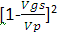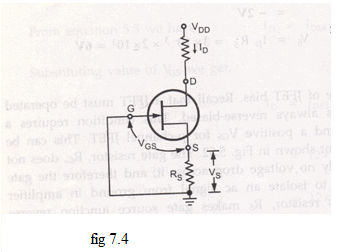VGS for N channel JFET is =-ID RS

Substuting  this value in the above equation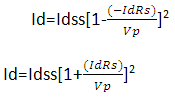For the N-chanel FET in the above figure

Is produces a voltage drop across Rs and makes the source positive w.r.t ground

in any JFET circuit all the source current passes through the device to drain circuit this is due to the fact that there is no significant gate current

therefore we can define source current as Is=Id and Vg=0 then

Vs= Is Rs =IdRs

Vgs=Vg-Vs=0-IdRs=-IdRs

Drawing the self bias line:-

Typical transfer characteristics for a self biased JFET are shown in the fig7.5.

The maximum drain current is 6mA and the gate source cut off voltage is -3V. This means the gate voltage has to be between 0 and -3V.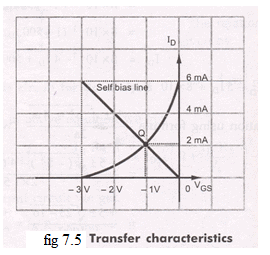Now using the equation VGS = -IDRS and assuming RS of any suitable value we can draw the self bias line.

Let us assume RS = 500Ω

With this Rs , we can plot two points corresponding to ID = 0 and Id = IDSS

for ID = 0

VGS = -ID RS

VGS = 0 X (500.Ω) = 0V

So the first point is (0 ,0)

( Id, VGS)

For ID= IDSS=6mA

VGS = (-6mA) (500 Ω) = -3V

So the 2nd  Point will be (6mA,-3V)

By plotting these two points, we can draw the straight line through the points. This line will intersect the transconductance curve and it is known as self bias line. The intersection point gives the operating point of the self bias JFET for the circuit.

At Q point , the ID is slightly  > than 2mA and VGS is slightly > -1V. The Q point for the self bias JFET depends on the value of Rs.If Rs is large, Q point far down on the transconductance curve ,ID is small, when Rs is small Q point is far up on the curve , ID is large.

VOLTAGE DIVIDER BIAS:-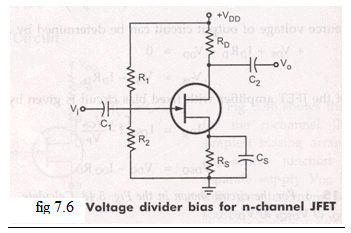The fig7.6 shows N channel JFET with voltage divider bias. The voltage at the source of JFET must be more positive than the voltage at the gate in order to keep the gate to source junction reverse biased. The source voltage is

VS = IDRS

The gate voltage is set by resistors R1 and R2 as expressed by the following equation using the voltage divider formula.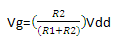For dc analysis fig 7.7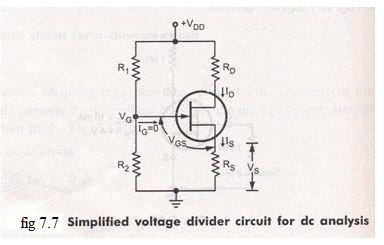Applying KVL to the input circuit

VG-VGS-VS =0

:: VGS = VG-Vs=VG-ISRS

VGS = VG-IDRS       :: IS = ID

Applying KVL to the input circuit we get

VDS+IDRD+VS-VDD =0

::VDS = V,-IDRD-IDR,

VDS = VDD-ID ( RD +RS )

The Q point of a JFET amplifier , using the voltage divider bias is

IDQ  = IDSS [1-VGS/VP]2

VDSQ = VDD-ID ( RD+RS )

Offer running on EduRev: Apply code STAYHOME200 to get INR 200 off on our premium plan EduRev Infinity!

,

,

,

,

,

,

,

,

,

,

,

,

,

,

,

,

,

,

,

,

,

;